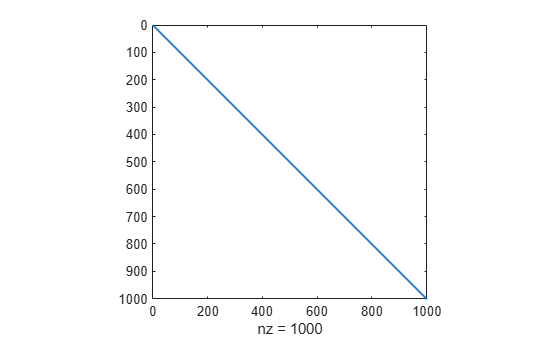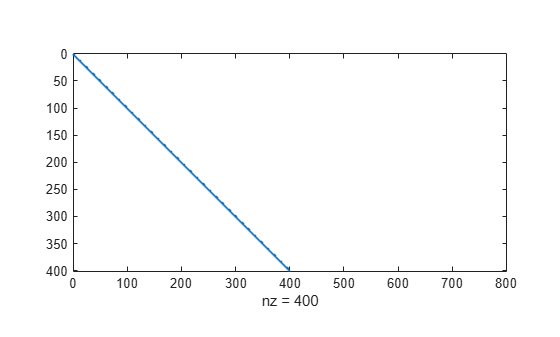Main Content

# speye

Sparse identity matrix

## Syntax

``S = speye``
``S = speye(n)``
``S = speye(n,m)``
``S = speye(sz)``

## Description

``S = speye` returns a sparse scalar `1`.`

example

````S = speye(n)` returns a sparse `n`-by-`n` identity matrix, with ones on the main diagonal and zeros elsewhere.```

example

````S = speye(n,m)` returns a sparse `n`-by-`m` matrix, with ones on the main diagonal and zeros elsewhere.```

example

````S = speye(sz)` returns a matrix with ones on the main diagonal and zeros elsewhere. The size vector `sz` defines `size(S)`. For example, `speye([2 3])` returns a 2-by-3 matrix.```

## Examples

collapse all

Create a 1000-by-1000 square sparse identity matrix and view the sparsity pattern.

```I = speye(1000); spy(I)```The result is the same as `sparse(eye(1000))`, but this latter version requires temporary storage for the full representation before it is converted to sparse storage.

Create a 400-by-800 sparse identity matrix and view the pattern of nonzeros.

```S = speye(400,800); spy(S)```This command is equivalent to `speye([400 800])`.

## Input Arguments

collapse all

Dimension sizes, specified as nonnegative integer scalars.

• If `n` is the only input argument, then `S` is an `n`-by-`n` identity matrix.

• If `n` or `m` is `0`, then `S` is an empty matrix.

• If `n` or `m` is negative, then it is treated as `0`.

Example: `speye(4)` creates a 4-by-4 identity matrix.

Example: `speye(3,6)` creates a 3-by-6 identity matrix.

Data Types: `single` | `double` | `int8` | `int16` | `int32` | `int64` | `uint8` | `uint16` | `uint32` | `uint64`

Dimension sizes, specified as a two-element row vector. The row vector is of the form `[numRows numCols]`.

• If an element of `sz` is `0`, then `S` is an empty matrix.

• If an element of `sz` is negative, then the element is treated as `0`.

Example: `speye([4 5])` creates a 4-by-5 matrix and is equivalent to `speye(4,5)`.

Data Types: `single` | `double` | `int8` | `int16` | `int32` | `int64` | `uint8` | `uint16` | `uint32` | `uint64`

## See Also

Introduced before R2006a

Download ebook